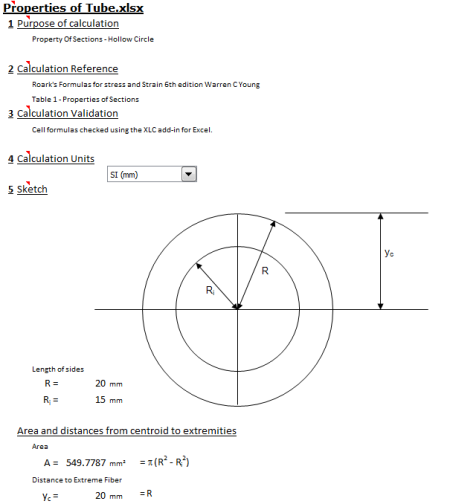Copy to Native Excel: Click in the text area below and copy. Then paste into cell A1 of a worksheet. All the formulas are brought in intact but Excel default behaviour controls formatting. A better transfer can be achieved using our XLC add-in for Excel (see below).
Length of sides
R = 20 mm in
Ri = 15 mm in
Area and distances from centroid to extremities
Area
A = =PI()*(D2^2-D3^2) mm² in²
Distance to Extreme Fiber
yc = =D2 mm in
Moments and products of inertia and radii of gyration about central axes
Moment of inertia
Ix = =(PI())/(4)*(D2^4-D3^4) mm⁴ in⁴
Iy = =(PI())/(4)*(D2^4-D3^4) mm⁴ in⁴
rx = =(1)/(2)*SQRT(D2^2+D3^2) mm in
ry = =(1)/(2)*SQRT(D2^2+D3^2) mm in
Plastic section moduli, shape factors, and locations of plastic neutral axes
Plastic section modulus
Zx = =1.333*(D2^3-D3^3) mm³ in³
Zy = =1.333*(D2^3-D3^3) mm³ in³
Shape factor
SFx = =1.698*((D2^4-D3^3*D2)/(D2^4-D3^4))
Copy to XLC Enhanced Excel: Click in the text area below and copy. Then use XLC’s ‘Web Paste’ button to paste at any position in worksheet. This method gives better format control, mathematical formatting and XLC equations.
Length of sides
R = 20 mm in
Ri = 15 mm in
Area and distances from centroid to extremities
Area
A = =PI()*(D2^2-D3^2) mm² in² =EQS(D7,"Units= ; EqnPrefix=Eqn. ; EqnNo= 0; Multiplication= 0; ShowWorking= 0; EqnStyle= 0; Eqp\$H\$31_0")
Distance to Extreme Fiber
yc = =D2 mm in =EQS(D9,"Units= ; EqnPrefix=Eqn. ; EqnNo= 0; Multiplication= 0; ShowWorking= 0; EqnStyle= 0; Eqp\$H\$33_0")
Moments and products of inertia and radii of gyration about central axes
Moment of inertia
Ix = =OVD(PI())/UND(4)*(D2^4-D3^4) mm⁴ in⁴ =EQS(D13,"Units= ; EqnPrefix=Eqn. ; EqnNo= 0; Multiplication= 0; ShowWorking= 0; EqnStyle= 0; Eqp\$H\$37_0")
Iy = =OVD(PI())/UND(4)*(D2^4-D3^4) mm⁴ in⁴ =EQS(D15,"Units= ; EqnPrefix=Eqn. ; EqnNo= 0; Multiplication= 0; ShowWorking= 0; EqnStyle= 0; Eqp\$H\$39_0")